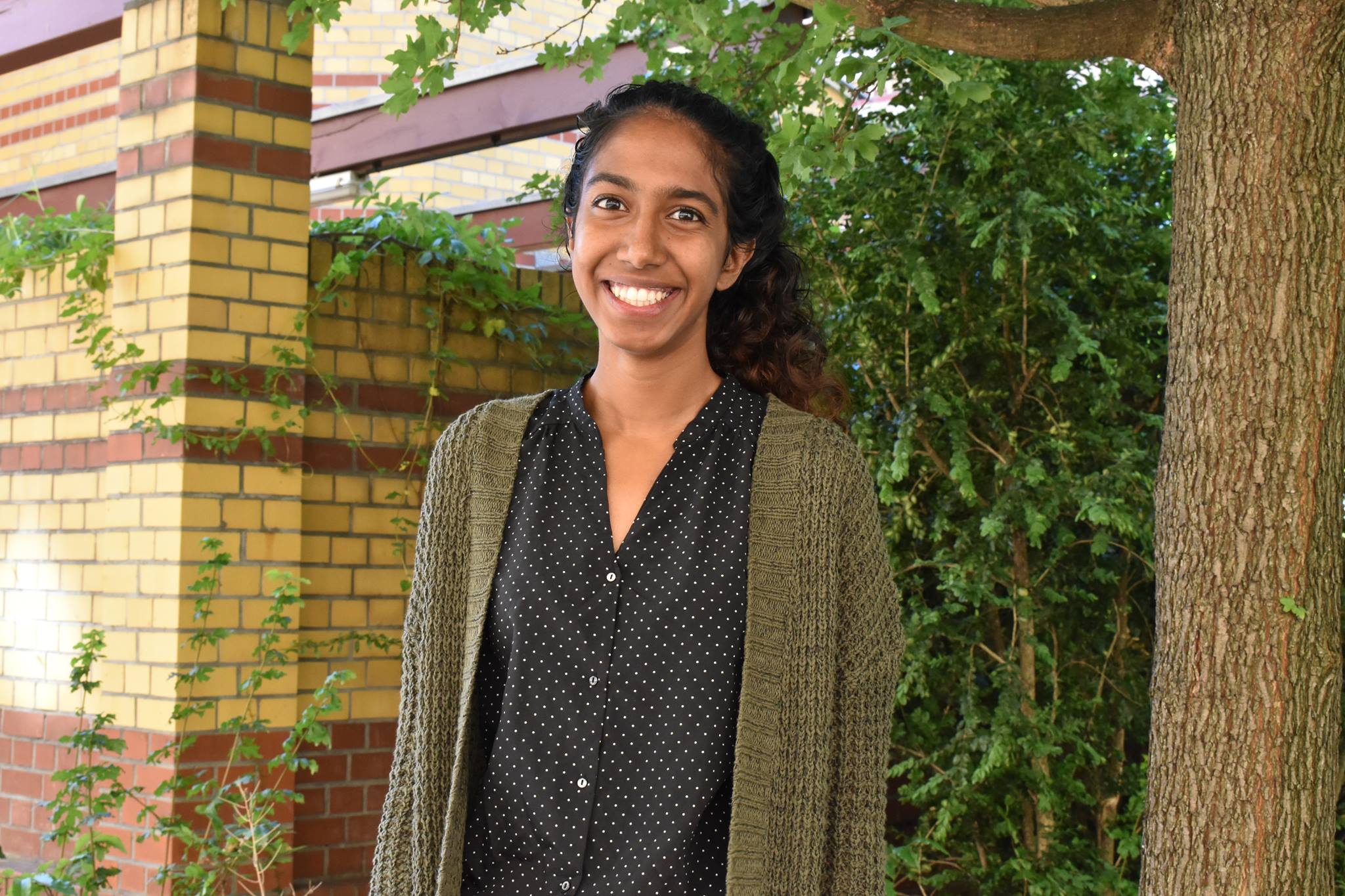# Sameera VemulapalliI am a fifth-year Ph.D. candidate in mathematics at Princeton University, advised by Manjul Bhargava. My email is sameerav@math.princeton.edu.

My primary research interest is arithmetic statistics, especially in shapes of orders and ideals in number fields (and their distributions). I am also interested in additive combinatorics and classical algebraic geometry. I like lattices, in pretty much any shape or form.

### Papers

• Bounds on Successive Minima of Orders in Number Fields and Scrollar Invariants of Curves, Preprint, arXiv:2207. 10522 [July 2022]
• Sumsets of sequences in abelian groups and flags in field extensions, Preprint, arXiv:2207. 10507 [July 2022]
• On intersections of symmetric determinantal varieties and theta characteristics of canonical curves, (joint with Avinash Kulkarni), Submitted , arXiv:2109.08740 [October 2021]
• Computing unit groups of curves, (joint with Justin Chen and Leon Zhang), Accepted by Journal of Symbolic Computation, arXiv:1808.02742 [Aug 2018]
• Uniform bounds for the number of rational points on symmetric squares of curves with low Mordell-Weil rank, (joint with Danielle Wang), Accepted by Acta Arithmetica, arXiv:1708.07057 [August 2017]

### Invited Talks

• Number Theory Seminar, Columbia University [April 2023]
• Arithmetic Statistics Special Session, AMS Sectional Meeting at University of Cincinnati [April 2023]
• Algebra and Number Theory Seminar, Emory University [March 2023]
• Number Theory Seminar, UC San Diego [February 2023]
• Algebra and Number Theory Seminar, Dartmouth College [February 2023]
• Number Theory Seminar, Princeton University/IAS [January 2023]
• Arithmetic Statistics Special Session, Joint Mathematics Meetings [January 2023]
• Palmetto Number Theory Series, University of South Carolina [December 2022]
• Algebra/Number Theory Seminar, Brown University [December 2022]
• Algebra, Geometry, and Number Theory Seminar, Tufts University: Bounds on Successive Minima of Orders in Number Fields [November 2022]
• Simons Foundation Conference, Geometry in Arithmetic Statistics, Schloss-Elmau, Germany: Bounds on Successive Minima of Orders in Number Fields and Scrollar Invariants of Curves [September 2022]
• SAXAG Seminar, Max Planck Institute for Mathematics in the Natural Sciences, Leipzig : On intersections of symmetric determinantal varieties and theta characteristics of canonical curves [July 2022]
• Nonlinear Algebra Seminar, Max Planck Institute for Mathematics in the Natural Sciences, Leipzig: An introduction to arithmetic statistics [June 2022]
• Adopha Seminar, Leiden University: Asymptotic bounds on successive minima of orders in number fields [January 2022]
• Seminar on Nonlinear Algebra, Max Planck Institute for Mathematics in the Natural Sciences, Leipzig: Cohen-Lenstra Heuristics [June 2019]
• Number Theory Seminar, University of Groningen: Rational points on symmetric squares of curves [July 2018]
• Seminar on Nonlinear Algebra, Max Planck Institute for Mathematics in the Natural Sciences, Leipzig: Counting Unit Groups of Curves [June 2018]

### Contributed Talks

• Arithmetic Statistics Seminar, Princeton University [November 2022]
• Graduate Student Seminar, Princeton University: Monogenic cubic rings [November 2022]
• Graduate Student Seminar, Princeton University: An introduction to sumset theorems: Kneser's theorem, Vosper's theorem, and generalizations [March 2022]
• Arithmetic Statistics Seminar, Princeton University: The Relationship between Segre cubic threefolds, Igusa quartic threefolds, and quintic and sextic etale algebras, P\ t. 2 [November 2021]
• Arithmetic Statistics Seminar, Princeton University: The Relationship between Segre cubic threefolds, Igusa quartic threefolds, and quintic and sextic etale algebras [November 2020]
• Arithmetic Statistics Seminar, Princeton University: The Relationship between Cayley cubic surfaces, Steiner quartic surfaces, and quartic etale algebras [November\ 2020]
• Arithmetic Statistics Seminar, Princeton University: The Relationship between Tricuspidal quartic plane curves, nodal cubic plane curves, and cubic etale algebras \ [November 2020]
• Graduate Student Seminar, Princeton University: Tricuspidal cubic plane curves and cubic etale algebras [November 2020]
• Arithmetic Statistics Student Seminar, Stanford University: Binary quartic forms having bounded invariants [June 2020]
• Princeton Open House Talk, Princeton University: Introduction to Arithmetic Statistics [March 2020]
• Arithmetic Statistics Student Seminar, Princeton University: Asymptotic bounds on successive minima of orders of number fields [December 2019]
• Computatational Tropical Geometry minisymposium, 2019 SIAM Conference on Applied Algebraic Geometry: Computing Unit Groups of Curves [July 2019]
• Graduate Student Seminar, Princeton University: Higher Composition Laws [April 2019]
• AMS Contributed Paper Session on Research in Geometry, Groups, and Representations by Undergraduate and Post-Baccalaureate Students, Joint Mathematics Meetings 2018: Uniform Bo\ unds for the Number of Rational Points on Symmetric Squares of Curves with Low Mordell-Weil Rank [January 2018]